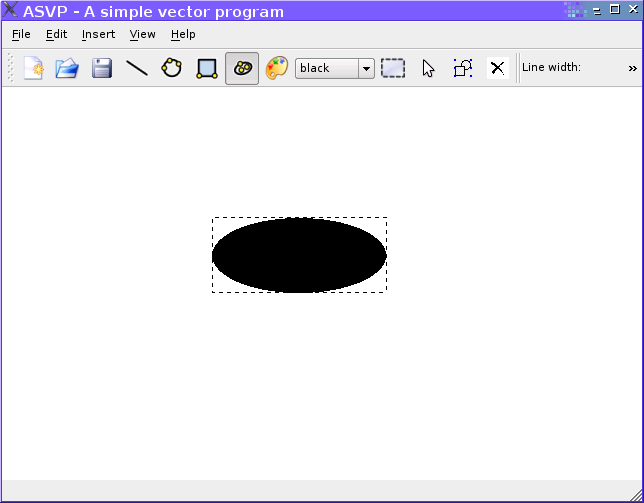# Rotation problems (object distortion)

Hi,
I’m writing a simple 2d program, but have problems rotating the primitives - for example my ellipse becomes a circle when the angle is 90 and at 180 it’s an ellipse again. I’ll attach 2 screenshots to illustrate the case.
Also here’s how I draw the ellipse and rotate it:

``````
void  Ellipse::draw()
{   // glPushMatrix();
glLineWidth(this->line_width);
const float DEG2RAD = 3.14159/180;
glColor3f(color,color,color);
glPushMatrix();
glTranslatef(p1,p1,0.0);
{ glTranslatef((p2-p1)/2.0,(p2-p1)/2.0,0.0);
glRotatef(rotationAngle,0,0,1);
glTranslatef(-(p2-p1)/2.0,-(p2-p1)/2.0,0.0);
}
if(scale!=1)
{
glScalef(scale,scale,scale);
}
glPolygonMode(GL_FRONT_AND_BACK, (isFilled)?GL_FILL:GL_LINE);
glBegin((isFilled)?GL_POLYGON:GL_LINE_LOOP);
for (int i=0; i < 360; i++)
{
}
cout << " is Filled: " << isFilled << endl;
glEnd();
``````

And here’s how I initialize the GL widget:

``````void NeHeWidget::initializeGL()
{
glClearColor(1,1,1,0.0);
glClear(GL_COLOR_BUFFER_BIT | GL_DEPTH_BUFFER_BIT);
glBlendFunc(GL_SRC_ALPHA,GL_ONE_MINUS_SRC_ALPHA);
}
void NeHeWidget::resizeGL( int width, int height )
{
glViewport(0,0,width,height);
glMatrixMode(GL_PROJECTION);
GLfloat x= (GLfloat) width/height;
glFrustum(-x,x,-1.0,1.0,1.0,1.0);
glMatrixMode(GL_MODELVIEW);
}
``````The following obvious bugs:

• in draw(), glPopMatrix is missing. This may lead to strange accumulations of matrices.
• in glFrustum, you have near==far. It is surprising to me that you see anything at all.

The first one might be the reason for the observed behaviour. Otherwise, I’d check how p1, p2 are changed in the program as you rotate the ellipse.

Well, I have glPopMatrix(), but it’s in the end of the function.
Also, I tried to change the glFrustum far and near, but couldn’t see anything after the changes, that’s why I set 1.0, 1.0 and it works.
Here’s the behaviour of p1 and p2. They don’t seem to change :
P1 -0.35625 P1 0.576531 P2 0.465625 P2 -0.214286
Rotation angle 10
is Filled: 1
P1 -0.35625 P1 0.576531 P2 0.465625 P2 -0.214286
Rotation angle 90
is Filled: 1
P1 -0.35625 P1 0.576531 P2 0.465625 P2 -0.214286
Rotation angle 180
is Filled: 1P1 -0.35625 P1 0.576531 P2 0.465625 P2 -0.214286
Rotation angle 10
is Filled: 1
P1 -0.35625 P1 0.576531 P2 0.465625 P2 -0.214286
Rotation angle 90
is Filled: 1
P1 -0.35625 P1 0.576531 P2 0.465625 P2 -0.214286
Rotation angle 180
is Filled: 1

And here’s the full code of the function:

``````void  Ellipse::draw()
{
glLineWidth(this->line_width);
const float DEG2RAD = 3.14159/180;
glColor3f(color,color,color);
glPushMatrix();
glTranslatef(p1,p1,0.0);
{ glTranslatef((p2-p1)/2.0,(p2-p1)/2.0,0.0);
glRotatef(rotationAngle,0,0,1);
glTranslatef(-(p2-p1)/2.0,-(p2-p1)/2.0,0.0);
}
if(scale!=1)
{
glScalef(scale,scale,scale);
}
glPolygonMode(GL_FRONT_AND_BACK, (isFilled)?GL_FILL:GL_LINE);
glBegin((isFilled)?GL_POLYGON:GL_LINE_LOOP);
for (int i=0; i < 360; i++)
{
}
cout << " is Filled: " << isFilled << endl;
cout << "P1 " << this->p1 << " ";
cout << "P1 " << this->p1 << " ";
cout << "P2 " << this->p2 << " ";
cout << "P2 " << this->p2 << " ";
glEnd();

if(this->isSelected())
{
glEnable (GL_LINE_STIPPLE);
glLineStipple (4, 0xAAAA);
glBegin (GL_LINE_STRIP);
glVertex2f(0,0);
glVertex2f(p2-p1,0);

glVertex2f(p2-p1,p2-p1);
glVertex2f(0,p2-p1);
glVertex2f(0,0);

glEnd ();
glDisable(GL_LINE_STIPPLE);
}
glPopMatrix();
}
``````

Could you try adding a glLoadIdentity() after glMatrixMode(GL_MODELVIEW)?

I added it, but it feels the same - there’s no change of any kind.

I guess it is due to the aspect ratio of your view. Did you tried other angles ? Did you tried other ellipsis ?

Also, don’t expect GL to explicitly transform the values of your vertices or so. It won’t.

But beforehand you should start from the basics, and specially about GL matrices: this code is somewhat pertining:

``````glTranslatef((p2-p1)/2.0,(p2-p1)/2.0,0.0);
glRotatef(rotationAngle,0,0,1);
glTranslatef(-(p2-p1)/2.0,-(p2-p1)/2.0,0.0);
``````

You don’t need to explicitly ‘untranslate’.

I can suggest Nehe’s tutorials or the opengl red book. That will definately help you understand the main principles, that will help you work with this library.

Hope that helps.